>

#### Chapter 2 Exponents of Real Numbers R.D. Sharma Solutions for Class 9th Math Exercise 2.1

Exercise 2.1

1. Simplify the following: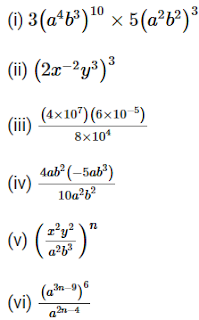Solution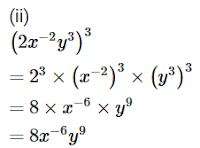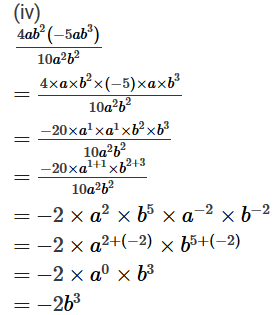2. If a = 3 and b = -2 , find the values of :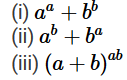Solution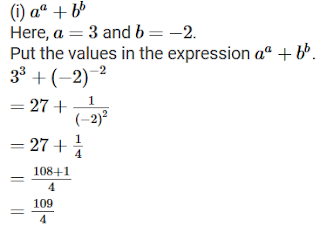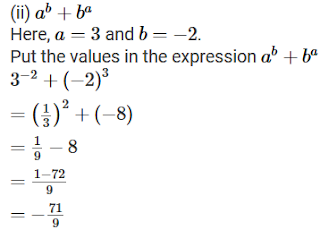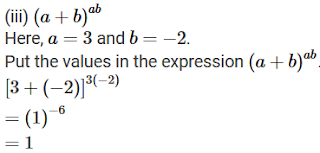3. Prove that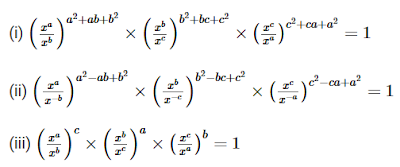Solution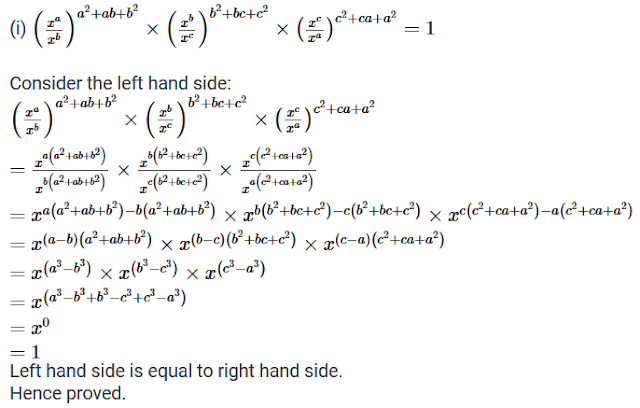4. Prove thatSolution(ii)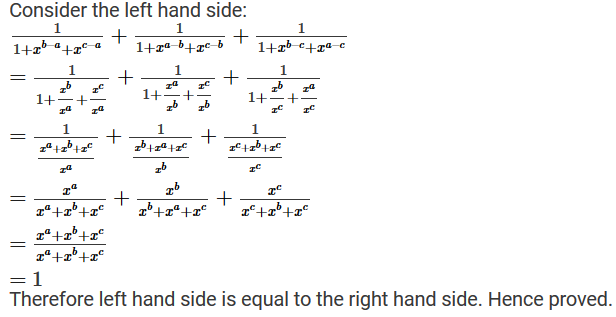5. Prove that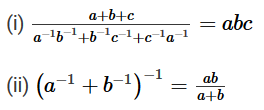Solution(ii)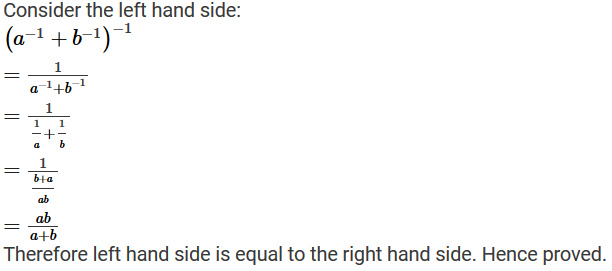6.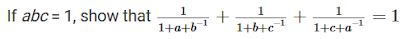Solution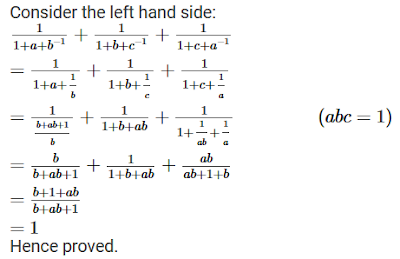7.  Simplify the followingSolution

(i)(ii)(iii)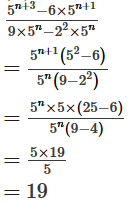(iv)Level - 2

8. Solve the following equation for x :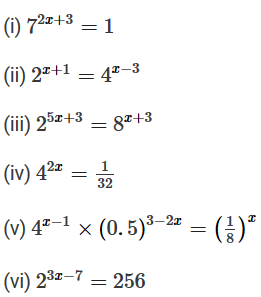Solution

(i)(ii)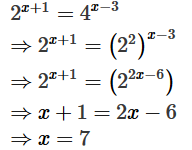(iii)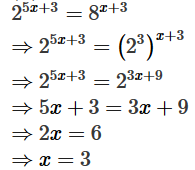(iv)(v)(vi)9. Solve the following equations for x :Solution

(i)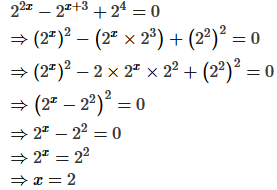(ii)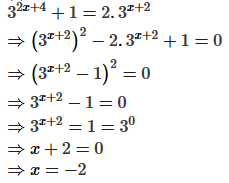10. If 49392 = a4b2c3, find the values of a,b and c, where a,b and c are different positive primes.

Solution

First find out the prime factorisation of 49392.11. If 1176 = 2a3b7c, find a,b and c.

Solution

First find out the prime factorisation of 1176.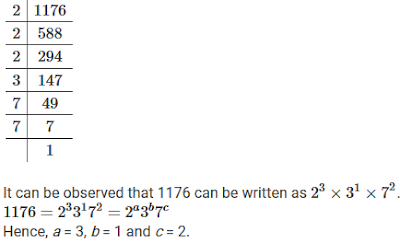12. Given 4725 = 3a5b7c, find
(i) The integral values of a,b and c
(ii) The values of 2-a3b7c

Solution13. If a = xyp-1, b = xyq-1 and c = xyr-1 prove that aq-rbr-pcp-q = 1

Solution